# Arbitrage Opportunities in Arbitrage

## Transcription

Arbitrage Opportunities in Arbitrage
```NBER WORKING
PAPER SERES
ARBITRAGE OPPORTUNITIES IN
ARBITRAGE-FREE MODELS OF
BOND PRICING
David Backus
Silverio Foresi
Stanley Zin
Working Paper 5638
NATIONAL
BUREAU OF ECONOMIC RESEARCH
1050 Massachusetts Avenue
Cambridge, MA 02138
June 1996
We thank Fischer Black for extensive comments; Jennifer Carpenter, Vladimir Finkelstein, and
Bruce Tuckman for guidance on industry practice; Anthony Lynch for pointing out an error in
an earlier draft; and Ned Elton for inadvertently initiating this project. Backus thanks the
National Science Foundation for financial support. This paper is part of NBER’s research
program in Asset Pricing. Any opinions expressed are those of the authors and not those of the
National Bureau of Economic Research.
O 1996 by David Backus, Silverio Foresi and Stanley Zin. All rights reserved. Short sections
of text, not to exceed two paragraphs, may be quoted without explicit permission provided that
full credit, including @ notice, is given to the source.
NBER Working Paper 5638
June 1996
ARBITRAGE OPPORTUN~IES
IN
ARBITRAGE-FREE MODELS OF
BOND PNCING
ABSTRACT
Mathematical
practitioners,
models
with practitioners
models to selected asset prices.
of bond pricing are used by both academics
introducing
time-dependent
parameters
and Wall Street
to fit “arbitrage-free”
We show, in a simple one-factor setting, that the ability of such
models to reproduce a subset of security prices need not extend to state-contingent
generally.
The popular Black-Derman-Toy
claims more
model, for example, overprices call options on long
bonds relative to those on short bonds when interest rates exhibit mean reversion.
We argue,
more generally, that the additional parameters of arbitrage-free models should be complemented
by close attention
to fundamentals,
which might include mean reversion,
multiple
factors,
stochastic volatility, and/or non-normal interest rate distributions.
David Backus
Stem School of Business
New York University
44 West 4th Street
New York, NY 10012-1126
and NBER
Stanley Zin
Graduate School of Industrial Administration
Carnegie Mellon University
Pittsburgh, PA 15213
and NBER
Silverio Foresi
Stem School of Business
New York University
44 West 4th Street
New York, NY 10012-1126
1
Introduction
Since Ho and Lee (1986) initiated work on “arbitrage-free”
academics and practitioners
have the same objective:
models of bond pricing,
have followed increasing] y divergent paths, Both groups
to extrapolate
from prices of a limited range of assets ~he
prices of a broacler class of state-contingent
claims. Academics
monious models, whose parameters are chosen to approximate
behavior
of interest rates and bond
models with more extensive
prices.
Practitioners,
sets of time-dependent
study relatively parsi“average”
or “typical”
on the other hand, use
parameters,
which they use to
Imatch current bond yields, and possibly other asset prices, exactly.
To practitioners,
the logic of this choice is clear: the parsimonious
models used by
academics are inadequate for practical use. The four parameters of the Vasicek ( 1977)
and Cox-Ingersoll-Ross
(1985) models, for example, can be chosen to match five points
on the yield curve (the four parameters plus the short rate), but do not reprodl~cr the
complete yield curve to the degree of accuracy required by market participants.
complex, multi-factor
accuracy.
Instead,
models cannot generally approximate
practitioners
Lee (1986) tradition,
including
Black and ]{arasinski
(1991),
rely almost universally
those developed
bond yields with sufficient
on models
by Black, Derman,
Cooley, LeRoy, and Parke (1992),
in the Ho and
and Toy (1990),
Heath, Jarrow, and
Morton (1992), Hull and White (1990, 1993), and many others, Although
approaches
Even
analytical
vary across firms and even within them, the Black- DernlaI1-Toy model is
currently close to an industry standard.
Conversely,
academics have sometimes expressed worry that the large parametf:r
sets of arbitrage-free
example is Dybvig
by repeated
models may mask problems with their structure.
A prominent
(1989), who noted that the changes in parameter values required
use of this procedure
contradicted
the presumption
of the theory that
the parameters are deterministic functions of time. Black and Karasinski (1991, p 57)
put it more colorfully:
“When we value the option, we are assuming that its volatility
is known and constant,
But a minute later, we start using a new volatility.
we can value fixed income securities by assuming we know the one-factor
process.
Similarly,
short-rate
A minute later, we start using a new process that is not consistent
the old one, ”
Dybvig
argued that these changes in parameter
with
values through time
implied that the framework itself was inappropriate.
We examine the practitioners’ procedure in a relatively simple theoretical setting,
a variant of Vasicek’s (1977) one-factor C,aussian interest rate model that we refer
to as the benchmark
theory.
Our thought experiment
is to apply models with time-
dependent drift and volatility parameters to asset prices generated by this theory. We
judge the models to be useful, in this setting, if they are able to reproduce
broad range of state-contingent
claims. This experiment
cannot tell us how well the
models do in practice, but it allows us to study the role of time-dependent
in an environment
that can be characterized
parameters
precisely. VVe find, in this environment,
that if the world exhibits mean reversion, then the use of time-dependent
in a model without
but cannot
mean reversion can reproduce
reproduce
these arbitrage-free
~models allow arbitrage opportunities:
model can be exploited
parameters
prices of a limited set of assets,
the prices of general state-contingent
say, the Black- Derman-Toy
prices of a
claims.
In this sense,
a trader basing prices on,
by a trader who knows the tr~~e
structure of the economy.
A striking exalmple of misprizing in this setting involves options on long bonds,
Options of this type vary across two dimensions of time: the expiration
option and the maturity of the bond on which the option is written.
date of the
The Blacl<-
Dermall-Toy
that can be
model has a one-dimensional
array of volatility parameters
chosen to reproduce either the prices of options with any expiration date on one-period
bonds or of options with common
expiration
dates on boncls of any maturity.
the world exhibits mean reversion, this vector cannot reproduce
array of prices of bond options.
of options on one-period
If the volatility parameters are chosen to match prices
bonds, then the model overprices options on long bonds.
While we think mean reversion is a useful feature
more general point:
But if
the two-dimensional
that misrepresentations
in a model,
of fundamentals,
it illustrates
a
whatever their form,
cannot generally be overcome by adding arrays of time-dependent parameters. From
this perspective, the role of academic research is to identify appropriate fundamentals,
which might include multiple factors, stochastic volatility, and/or
rate movements.
We conjecture
that arbitrage-free
no~l-uormal interest
models with inaccuracies
along
any of these dimensions will misprice some assets as a result.
2
A Theoretical
We use a one-factor
the practitioners’
pricing
model
Benchmark
Gaussian interest rate model as a laboratory
procedure
to observed
of choosing
asset prices.
in which to examine
time-dependent
parameters to fit a bond
The moclel is a close relative of Vasicek
(1977). Although in some respects it is simpler than those used by practitioners, its
log-linear structure is extremely useful in clarifying the roles played by its various
parameters.
2
To fix the notation,
let ~ be the price at date t of a zero-coupon
71,the claim to one dollar at date t + TL,By convention
one dollar).
bond of maturity
fi~= 1 (one dollar today costs
Bond yields are
y;
=
–n-l
log
b;
and forward rates are
f:
We label the short
(1)
= log(b;/b;+’).
rate rt = Y; = f:.
We characterize asset prices in our benchmark theory, or laboratory,
kerTLel: a stochastic
process governing prices of state-contingent
such a process is guaranteed in any arbitrage-free
environment.
with a prici~~g
claims. Existence of
We describe the kernel
for our theoretical environment in two steps. The first step involves an abstract state
variable z, whose dynamics follow
~t+l
=
—
with {Et} distributed
The parameter
pzt + (1 – p)6+
Zt +(1
normally
&t+l
and independently
y controls mean reversion:
with mean zero and variance az.
with y = 1 the state follows a random
walk, but with values between zero and one the conditional
z converges
to the unconditional
(2)
~t) +Et+l,
—p)(J—
mean of future values of
mean 6. Step two is the pricing kernel nt, which
satisfies
– log ~n~+~=
The parameter
z~ +
~E~+I
A, which we refer to as the price of risk, determines
between innovations
(:3)
.
the covariance
to the kernel and the state and thus the risk characteristics
of
bonds and related assets.
Given a pricing kernel, we derive prices of assets from the pricing relation
(4)
1 = Et(m~+~Rt+l),
which holds for the gross return Rt+l on any traded asset. Since the one-period
on an 71+ l-period
retllrn
bond is ~+1 /b~+l, the pricing relation gives us
b:+l = Et(?7~t+l~;+l)7
(5)
which allows us to compute bond prices recursively, starting with the initial condition
b:=l.
Consider a one-period
bond.
From equation
we see that the price is the conditional
(5) and the initial condition
mean of the pricing kernel:
3
L? = 1
b: = Elnl,t+l.
Since the kernel is conditionally
normal random
log E(x)
variables:
= p + 02/2.
–zt and conditional
lognormal,
we need the following
if log x is normal
From equation
property
with mean p and variance
(3) we see that log ?n~+l has conditional
variance (Aa)2. Thus the one-period
of log-
az, then
mean
bond price satisfies
log b; = –2, + (Aa)2/2
and the short rate is
r~ = –logb;
Thus the short rate ~ is the state z with a shift of origin.
6 – (Ao)2/2,
(6)
= z~ – (Aa)2/2.
The mean short rate is
which we denote by L in the rest of the paper.
The stochastic
process for z, equation
(2), implies similar behavior
for the short
rate:
rt+l = Tt +(1
–~)(p–
Tt) +
Et+l,
a discrete time version of Vasicek’s (1977) short rate diffusion.
short rate are
for n >1,
Future values of ~;~
n
which yields conditional
E,(r,+n)
and
first and second moments of
= l-, + (1 – 9“)(P
– ~t)
(8)
w
(9)
j=l
We return to these formulas later.
Prices of long bonds follow from (5); details are provided in Appendix A.1. Their
are conveniently summarized by forward rates, which are linear functions
properties
of the short rate:
f;=rt+(l
–w’’)(p–rt)+
A2–
[
(
A+
1;:)2]a,2,
(lo)
for all T1~ O. Given forward rates, we can compute bond prices and yields from their
definitions, The right side of equation (10) has a relatively simple interpretation,
If
we compare it to (8), we see that the first two terms are the expected short rate r~
periods in the future. We refer to the last term as a risk premium and note that it
4
depends on three parameters:
the magnitucleof
risk (o),
the price of risk (A), and
mean reversion (y).
Both the Ho and Lee (1986)
capable of reproducing
and Black,
Derman,
and Toy (1990)
an arbitrary forward rate curve (equivalently,
models
are
yields or bond
prices), including one generated by a theoretical model like this one, An issue we
address later is whether this capability extends to more complex assets, With this
in mind, consider a European call option at date t, with expiration date t + ~ and
Given the
bond with maturity n at expiration.
of bond prices in this setting, the call price is given by the Blacl<-Sc.holes
strike price k, on a zero-coupon
lognormality
(1973) formula,
T,n
Ct
where N is the cumulative
= b;+”N(dl)
– kb:fv(~2),
normal distribution
~
_
log[b:+n/(b:k)]
(11)
function,
+ v;,n/2
l—
and the option
volatility
is
(12)
See Appendix
A.4. .Jamshidian (1989) reports a similar formula for a continuous-ti]ne
version of the Vasicek model.
of the Black-Scholes
3
~ in ( 12).
Values
in this paper,
to make a theoretical
illustrate the theory
with numerical
an informal
matching
moment
applications
formula is the role of the mean reversion parameter
Parameter
We are trying,
The primary difference from conventional
examples.
point,
but we find it useful to
The parameter
exercise based on properties
values come from
of monthly
yields for
[JS government securities computed by McCullocb and Kwon ( 1993). Some of the
properties of these yields are reported in Table 1 for the sample period 1982-91,
We choose the parameters to approximate some of the salient features of bond
yields using a time interval of one month. From equation (7) we see that ~ is the
unconditional
mean of the short rate, so we set it equal to the sample mean of the
5
one-month
yield in Table 1, 7.483/1200.
(The 1200 converts an annual percentage
rate to a monthly yield. ) The mean reversion parameter y is the first autocorrelation
of the short rate.
In Table 1 the autocorrelation
is 0,906, so we set y equal to this
value. This indicates a high degree of persistence in the short rate, but less than with
a random walk. The volatility parameter
a is the standard deviation
of innovations
to the short rate, which we estimate with the standard error of the linear regression
(7). The result is a = 0.6164/1200.
the mean, standard deviation,
final parameter,
Thus the values of (p, O, p) are chosen to match
and autocorrelation
of the short rate. We choose the
the price of risk A, to approximate
the slope of the yield curve. Note
from (10) that mean forward rates, in the theory, are
This tells us that to produce
an increasing
mean forward
increasing mean yield curve) we need A to be negative.
rate curve (implying
an
The price of risk parameter,
in other words, governs the average slope of the yield curve. One way of fixing A, thent
is to select a value that makes the theoretical mean yield curve similar to the sample
mean yield curve, given our chosen values for the other three parameters.
An example
is pictured in Figure 1, where ~vesee that A = —750 produces theoretical
mean yields
(the line in the figure) close to their sample means (the stars) for maturities between
one month and ten years. With more negative values the mean yield curve is steeper,
and with less negative
(or positive)
values the yield curve is flatter (or downward
sloping).
Thus we see that all four parameters
dynamics
benchmark
4
are required for the theory to imitate the
of interest rates and the average slope of the yielcl curve.
We use these
values later to illustrate differences in prices across bond pricing models.
Ho and Lee Revisited
We turn now to the use of time-dependent
parameters
to fit theoretical
models to
observed asset prices. We apply, in turn, analogs of the models of Ho and Lee ( 1986)
and Black, Derman, and Toy (1990) to a world governed by the benchmark
theory of
Section 2.
Our first example is a (;aussian analog of Ho and Lee’s (1986) binomial
rate model. The analog starts with a state equation,
6
interest
with time-dependent
innovations
parameters
{CYt} and normally
and independently
distributed
{qt} with mean zero and constant variance 92. The pricing kernel is
– log T?lt+l = ~t + 7TL+1.
(14)
lVe use different letters for the parameters than in the benchmark theory to indicate
that they may (but need not ) take on different values, Our Ho and Lee analog differs
from the benchmark
exhibit
theory in two respects,
First, the short rate process does not
mean reversion,
which we might think of as setting ~ = 1 in equation (2).
“drift” parameters {at}.
Second, the state equation (13) includes time-dependent
Given equations
(13,14),
the pricing relation (5) implies a short rate
and forward rates
(15)
j=l
for rZ~ 1. See Appendix
A.2,
Note that (10) differs from (15) of the benchmark
in
two ways. One is the impact of the short rate on long forward rates. A unit increase
in r is associated
benchmark.
with increases in f“
of one in Ho and Lee, but (1 — pn) < 1 in the
The other is the risk premium, the final term in ecluation (1,5).
Despite these differences, time-dependent drift parameters allow the Ho and Lee
model to reproduce some of the features of the benchmark theory. One such feature
is the conditional
mean of future short rates. The future short rates implied by this
model are
.
which implies conditional
means of
71
Et(rt+n)
for 71>0.
equation
= ~t + ~
~t+j
j=l
If we compare this to the analogous expression for the benchmark
(17)
theory,
(8), we see that the two are equivalent if we set
n
(18)
7
Thus the time-dependent
imitate this consequence
drift parameters of the Ho and Lee model can be chosen to
of mean reversion in the benchmark
In practice it is more common
theory.
to use the drift parameters to fit the model to the
current yield curve. To fit forward rates generated by the benchmark
theory we need
[compare (10,15)]
5 at+j
=
(1 -~’’)(p
-r,)
+ [A’ - (A+(l
-~’L)/(l
-~))’]
cJ2/2
j=l
- [72 - (7+
(19)
~)’] P2/2
The drift parameters implied by (18) and (19) are, in general, different.
we can equate the two expressions
Since
when y = 1 by setting ~ = a and ~ = J. But
when O < v < 1 the two expressions cannot be reconciled.
where we graph the two choices of cumulative
This is evident in Figure 2,
clrift parameters,
~~=1 a~+j, using the
parameters estimated in Section 3, with ~ = a, ~ = A, and r = 3.0/1200.
parameters
that reproduce
the conditional
mean converge
rapidly
The drift
as the effects of
mean reversion wear off. But the drift parameters that fit the current yield curve get
steadily smaller as they offset the impact of maturity
model.
This results, for the range of maturities
structure of expected
The discrepancy
on the risk premium
future short rates, while the benchmark
in the figure between
in this
in the figure, in a declining
term
implies the reverse,
the two choices of drift parameters
is a
concrete example of
Remark
current
ge~lerally
1 The parameters
yield
curve,
of
the Ho
or the conditio~tal
and Lee
means
model
of future
can
short
be chose~t
ratesj
the
ca~l~lot
do both.
This property of the Ho and Lee model is a hint that time-dependent
do not adequately
Dybvig
to match
bllt they
capture
drift parameters
the effects of mean reversion in the benchmark
(1989, p ,5) makes a similar observation.
A closer look indicates
theory.
that the
difficulty lies in the nonlinear interaction in the risk premium between mean reversion
and the price of risk.
In the benchmark
forward rate [see equation
theory, the risk premium
(10)] is
8
on the ~~-period
In the Ho and Lee model, equation
(15), the analogous expression is
If we choose ~ = A and ~ = a the two expressions are equal for ~1= 1, but they move
apart as 71grows. The discrepancy
A similar comparison
conditional
noted in Remark 1 is a direct consequence.
of conditional
variances
also shows signs of strain.
The
variances of future short rates implied by the Ho and Lee model are
Vart(rt+m)
= n~z.
If we compare this to the analogous expression in the benchmark theory, equation (9),
we see that they generally differ when ~ # 1. With y < 1 and ~ = CJ,the conditional
variances are the same for n = 1, but for longer time horizons they are greater in the
Ho and Lee model.
Remark
We summarize this discrepancy
2 The parameters
the conditional
variances
in
of the Ho and L~e model
of future
Thus we see that additional
short
cannot
be chosc:u
to re])roduce
rates.
drift parameters
allow the Ho and Lee model
to
imitate some of the effects of mean reversion on bond yields. They cannot? however,
reproduce
the conditional
summarized
this feature
variances of the benchmark
of the Ho and Lee model
and Lee model starts with an unreasonable
theory,
Dybvig
more aggressively:
implicit assumption
(1989, p 5)
“[T]he
Ho
about innovations
in
interest ratesj but can obtain a sensible initial yield curve by making an unreasonable
assumption
about expected
interest rates. lJnfortunately,
while this ,.. give[s] correct
pricing of discount boncls..., there is every reason to believe that it will give illcorrert
pricing of interest rate options. ” Dybvig’s
intuition about options is easily verified.
The Black- Scholes formula, equation (11), applies to the Ho and Lee model if we use
option volatility
(20)
v; ~ = Var,(log b~+~) = ~(n~)’.
This formula cannot be reconciled
for all combinations
~ to reproduce
with that of the benchmark
theory, equation
( 12),
of ~ and n unless y = 1. If we choose the volatility parameter
the option
we overstate the volatilities
volatility
ZJI,Iof a short option
of long options
on long bonds.
on a short bond,
then
As a result, the model
overvalues options with more clistant expiration dates ancl/or longer underlying bonds.
We see in Figure 3 that call prices CTI1implied by the Ho and Lee model can
be substantially
higher than those generated
9
by the benchmark
theory.
The figure
expresses the rnispricing as a premium of the Ho and Lee price over the price generated
by the benchmark
theory.
Benchmark
prices are based on the parameter
values of
Section 3. Ho and Lee prices are based on drift parameters that match current bond
prices, equation
volatility
(19),
and a volatility
VI,1 of a one-period
p = a that Imatcbes the option
on a one-period
bond.
Both are evaluated
at
For ~ = 1 the two models generate the same call price, but
strike price k = bj/bJ+”.
for options
option
parameter
with expiration
dates 12 months in the future the Ho and Lee price is
more than ,50 percent higher.
5
Black,
Derman,
and Toy Revisited
Black, Derrnan, and Toy ( 1990) extend the time-dependent
Lee to a second dimension.
logarithm
parameters
They base bond pricing on a binomial
of the short rate in which both drift ancl volatility
process for the
are tilne-(le~]elldetlt.
We build an analog of their model that retains the linear, Gaussian
previous sections, but includes these two sets of time-dependent
a two-parameter
distribution
like the normal, time-dependent
be used to match the conditional
to mitigate the tendency
distribution
of Ho and
structure
parameters.
of
Given
drift and volatility can
of future short rates exactly and thus
of the Ho and Lee model to overprice
question is whether they also allow us to reproduce
long options.
The
the prices of other interest-rate
derivative securities.
Our analog of the Black-Derman-Toy
model adds time-dependent
structure of the previous section: a random variable z follows
Zt+l
=
~t +
~t+l
volatility to the
(21)
+ qt+l
and the pricing kernel is
– log ?n~+~= z~ + yqt+~ .
The new ingredient
is that each qt has time-dependent
differs from the benchmark
(22)
variance ~~~. This structure
theory in its absence of mean reversion (the coefficient of
one on zt in the state equation)
and in its time-dependent
drift (the CY’S)and volatility
(the ~’s).
We approach
rate is
bond pricing as before.
We show in Appendix
r~ = – log (EtT?z~+~)= z~ – (~pt+~)2/2
10
A.2 that the short
and forward rates are
for 71~ 1. Equation
(23) reduces to the Ho and Lee expression,
equation
(15), when
@t = @ for all t. AS in the Ho and Lee model, forward rates differ from the benchmark
in both the impact of short rate movements on long forward rates and the form of
the risk premium.
We can use both sets of time-dependent
in the benchmark
theory.
parameters
Consider the conditional
to approximate
distribution
asset prices
of future short rates.
The short rate follows
so future short rates are
Their conditional
mean and variance are
Et(rt+n)
= ~t +
72(P?+l
– P:+,z+l
)/2
+fat+,
j=l
This model, in contrast
of the benchmark
to Ho and Lee, is able to match the conditional
theory, which we do by choosing volatility parameters
variances
that decline
geometrically:
This implies conditional
variances of
the same as ecluation (9) of the theory. Similar patterns of declining time-dependent
volatilities are common when these methods are used in practice, Black, Derman, and
11
Toy’s numerical example included (see their Table I). To match the conclit ional mean
of the benchmark
theory, equation
Thus we see, as Black, Derman,
(8), we choose drift parameters
that satisfy
and Toy (1990, p :33) suggest, that we can fit the
first two moments of the short rate with two “arrays” of parameters:
Remark
3 The parameters
duce the conditional
means
of the Black-Derman-Toy
and variances
of future
model
short
caTl bc chosen
to repro-
rates.
Given the critical role played by volatility in pricing derivative assets, this represents
an essential advance beyond Ho and Lee.
Despite time-dependent
reproduce
the conditional
benchmark
equation
theory.
volatility
moments
parameters,
the model cannot simultaneo~lsly
of the short rate and the forward
The drift parameters
that reproduce
rates of the
the forwarcl rate curve,
(10), are
=
(1 - pn)(p - r,)+
[A’ -(A+
(1 - y“)/(1
- y))’]
0’/2
j=]
(28) and (29) we see that the two are not equivalent, in general. ii~e note
Comparing
the difference in
Remark
4 Give71 volatility
of future
rates,
to match
the current
they
cannot
parameters
the drift parameters
generally
yield
curve,
(27)
that reproduce
the conditional
of the Black- Derman-Toy
or the conditioltal
means
model
of future
variaTlces
can be choserl
short
rate.~, but
do both.
Figure 4 plots the difference between (29) and (28). The parameter values are those
of Section 3, with ~ = A, p~+j = y ~–la, and r = ;3.()/12()(). The differences are smaller
than those of Figure 2 for the Ho and Lee model, but are nonzero nonetheless.
Remark 4 is a hint that the Black-Dermall-Toy
prices for the full range of state-contingent
analog cannot generate accurate
claims in the benchmark
12
economy, but we
can see this more clearly by looking at specific assets. Consider European call options
on zero-coupon
bonds.
Once more the lognormal
the Black- Scholes formula, equation
structure of the model means that
(11), applies. If we choose drift parameters to fit
the current yield curve, then any difference between call prices in the model and the
benchmark
theory must lie in their option volatilities.
Black-Derman-Toy
The option volatility for the
analog is
V;,l=
n2 ~‘
j=l
~~+j.
Appendix A.4. If we restrict ourselves to options on one-period bonds (so that
71= 1), we can reproduce the volatilities of our theoretical environment by choosing
see
~6’s that decline geometrically
with time, the same choice that replicates COI]ditional
variances of future short rates, equation
(27).
However, the Black- Dermal~-Toy analog cannot simultaneously
of options on bonds of longer maturities.
rameters of equation
reproduce
If we use the geometrically
prices
declining pa-
(27), the option volatility is
From (12) we see that the ratio of option volatilities is
BDT Volatility
Benchmark
nz
Volatility
= (1 + ~ + .
This ratio is greater than one when O < w <1
Blacl~-Dernlall-Toy
analog prices options
options on long bonds.
+ ~“-l)z
and n > 1, and implies that when the
on short bonds
correctly,
it will overprice
We see in Figure 5 that this rnispricing gets worse the longer
the maturity of the bond, and is greater than 150 percent for bonds with maturities
of two years or more. As in Figure 3, benchmark
of Section
reproduce
3, the drift parameters
prices are based on the parameters
of the Black- Dernlall-Toy
analog are chosen to
the current yield curve, the strike price is k = b~/b~+”, and the expiration
period is r = 6. Thus we have
Remark
prices
5 The parameters
of call options
The difference
of the
o~t bonds for
in option
Black-Derman-Toy
all maturities
volatilities,
model
cannot
a~ld expiration
dates.
and hence in call prices, between
models stems from two distinct roles played by mean reversion in determining
13
the two
prices
of long bonds.
Mean reversion appears, first, in the impact of short rate innovations
on future short rates. We see from (9) that the impact of innovations on future short,
rates, in the theory, decays geometrically
with the time horizon
easily mimicked in the Black-Dernlan-Toy
parameters
concerns
{~f}
that decay at the same rate.
the impact
Dermall-Toy
~, This feature is
model, as we have seen, by using volatility
The second
of short rate movements
role of mean reversion
on long bond prices.
In the Black-
model, a unit decrease in the short rate results in an 71unit, increase in
the logarithm of the price of an ~~-period bond; see Appendix
theory, the logarithm of the bond price rises by only (I+p+.
y); see Appendix
Al.
.4.2. In the benchmark
~.+p’’-’)
= (1–p’’)/(l
–
This attenuation of the impact of short rate innovations on long
bond prices is a direct consequence
by choosing geometrically
of mean reversion.
call prices generated by the Black- Derman-Toy
the Black- Derman-Toy
It is not, however, reproduced
declining volatility parameters and is therefore missing from
analog.
analog does not reproduce
Stated somewhat differently:
the hedge ratios of the benchmarl{
theory.
As a practical matter, then, we might expect the Blat.k-Dernlall-Toy
work well in pricing options on short term instruments,
For options on long bonds,
hence the call price.
This procedure
procedure
however, the model overstates the option volatility
A common
example of such an instrument
and
is a callable bond.
will generally overstate the value of the call provision
in the theoretical environment,
to
including interest rate caps.
to the issuer,
and thus understate the value of a callable long-term
bond.
The discrepancy
between option prices generated
by the benchmark
the Black-Dernlan-Toy
analog illustrates one difficulty
dependent
that a one-dimensional
parameters:
parameters cannot reproduce
the conditional
vector of time-dependent
volatility
variances of bond prices across the two
dimensions of maturity and time-to-expiration.
misprizing:
theory and
of using models with tinle-
We turn now to a second example of
a class of “exotic.” derivatives whose returns display different sensitivity
than bonds to interest rate movements,
Consider an asset that delivers the power O of the price of an n-period
period in the future.
sensitivity
to interest rate movements
the convenient
log-linearity
made popular
=
- (.,+
by Bankers Trust, yet ret sins
of bond prices in our framework.
compare the prices implied by the benchmark
log ~
bond one
This asset has some of the flavor of derivatives with magnified
(Aa)2/2)
As with options,
we
theory,
+Hlogb;+
(’+’11::)2a2’2
–@(l–pn)(p–
r,),
14
(30)
with those generated by the Black- Derman-Toy
analog,
n
–6’ ~
[(n– j)(~t+j+l
– Qt+j ) -(~+
n - j)2(P?+j+l
-
Pt+j)2/2]
-
(31)
j=l
Both follow from pricing relation (4) with the appropriate
Suppose
we choose
the parameters
pricing kernel.
of the Black-Dernlall-Toy
analog
to ulatch
the conditional variance of future short rates [equation (26)], the current, yield curve
[equation (29)], and the price of risk [y = A]. ~~an this model reproduce the prices of
our exotic asset for all values of the sensitivity parameter 9? The answer is generally
no. When n = 1 the two models generate the same price d) for all values of H,just as
we saw that the two sets of cumulative
drift parameters were initially the same (see
Figure 4 and the discussion following Remark 4). For longer maturities, however, the
prices are generally different.
Figure 6 is an example with 7Z= 60 and r = ~3.00/1200.
We see that for values of O outside the unit interval, the Black-Dermall-Toy
overprices
the exotic,
options on long bonds.
Remark
bonds,
yield
Dermall-Toy
curve
but they cannot
These examples
the difference
of the Black-Derman-Toy
and the term
generally
structure
replicate
models
is smaller than for
model
can be chosrn
of volatility
the prices
for
of more
of rnispricing illustrate a more general result:
options
exotic
which define n-period-ahead
analog implies
15
ort
onc-
derivatives.
theory.
This
factors,
state prices. We show in the Appendix
theory implies discount factors
and the Black- Derman-Toy
discount
to match
that the Blacl<-
analog cannot replicate the state prices of the benchmark
result is stated most clearly using stochastic
ark
between
Thus
6 The parameters
both the current
period
although
analog
that the benchm-
As long as y # 1 and a # O, the seconcl expression cannot be made e(luiva]ent to the
first:
Proposition
ma?t-Toy
benchmark
1 The time-depende.?lt
model
cannot
be chosen
dTift
an,d volatility
to reproduce
discount
factors
of th~
theory.
A proof is given in Appendix
A.3. The difficulty lies in the innovations,
There is no choice of volatility parameters {@t} in Blaclt-Dernlall
cate the nonlinear interaction
benchmark
of th~ Black-Dcr-
parameters
the stochastic
-Toy that can repli-
of mean reversion (y) and the price of risk (A) in the
theory.
In short, the time-dependent
Black-Derman-Toy
drift and volatility
parameters
of a model like the
analog cannot replicate the prices of derivative
assets generated
by a model with mean reversion.
6
Arbitrage
In the laboratory
and Profit Opportunities
of the benchmark
theory, the Black-Dermall-Toy
analog systenlati-
cally misprices some assets. In this section we consider strategies that might be used
to profit from traders using prices indicated
by the Black-Dermall-Toy
model.
One way to exploit someone trading at Black-Derman-Toy
prices is to arbitrage
with someone trading at benchmark prices. If, as we have seen, a Black- Dern~allToy trader overprices
benchmark
options
on long bonds relative to those on short rate, and a
trader does not, then we can buy frolm the latter and sell to the former,
thus making a riskfree profit. This is as clear an example of arbitrage as there is. Bllt
since such price differences are so obvious,
they are unlikely to be very common.
It is less easy to exploit a Black-Derman-Toy
at prices dictated by the model.
Since Black- Derman-Toy
a pricing kernel, they preclude riskless arbitrage.
namic strategies
trader when trades take place only
prices are consistent with
We can often devise, however, dY-
whose returns are large relative to their risk.
16
One sllch strategy
involves call options on long bonds, which (again) the Black- Dernlall-Toy
erally overvalues.
we might expect to make a profit. This seems particularly
period,
model gel]-
If we sell the option to the trader at date t, and liquidate at t + 1,
since the option is overvalued
likely in the option’s
final
but the bonds on which the option is written
are not.
Before we evaluate this strategy, we need to explain how the Blacl~-Dernlal] -Toy
model gel]trader prices assets through time. .4 trader using the Black-Dermall-Toy
erally finds, both in practice and in our theoretical
parameters
must be reset each period.
setting, that the t,iI~le-del]ell(iellt
In our setting,
suppose
the trader chooses
volatility parameters at date t to fit implied volatilities from options on short bonds.
As we have seen, this leads her to set {~~~+1,~t+z, ~t+3, . .} eclual to {a, pa, y20, . . .}.
If the parameters were literally time-dependent,
then in the following period logic dic-
tates that we set {~~+2, ~t+3, /~~+4,. . .} equal to {pa, V2a, W30, . .}. But if we calibrate
once more to options on one-period bonds, we would instead use {cJ, pa, ~2a,
.}.
This leads to a predictable
upward jump in the volatility
parameters
riod to the next, as in Figure 7. Similarly, the drift parameters
each period to retain the model’s
changes in parameter
ability to reproduce
from one pe-
must be adjusted
the current yield curve, These
values through time are an indication
that the model is im-
perfectly imitating the process generating state prices, but they nevertheless improve
its performance.
In this sense the internal inconsistency
noted by Dybvig (1989) is a
symptom of underlying problems, but not a problem in its own right. Thus our trader
prices call options using drift and volatility parameters that match, each period, the
yield curve and the term structure of volatilities
bonds, equations
impliecl by options
011
one-period
(29) and (27), respectively.
Now consider a strategy against such a trader of selling a ~-period
~t-period bond and buying it back one period later. The gross one-period
option on an
return from
this strategy is
Rt+l
=
–c;;;’’’/c;’n,
for r z 1, with the convention that an option at expiration has value c~)” = (~ – k)+.
The profit from this strategy is not riskfree, but it can be large relative to the risk
involved.
In the world of the benchmark
theory, the appropriate
adjustment
is given by the pricing relation (4). We measure the mean excess risl{-adjusted
for risk
return
by
a = E (7nt+l Rt+l)
using the pricing kernel nt for the benchmark
— 1,
economy.
This measure is analogolls to
Jensen’s alpha for the CAPNI and is the appropriate risk adjustment in this setting.
For the trading strategy just described, and the parameter values of Section :3, the
returns are in the neighborhood
of several hundred percent per year; see Figurt~ 8.
17
The returns in the figure were computed
(~=2)
by .Monte Carlo, and concern
options on bonds for maturities (n) between 1 and 24 months.
greater with ~ = 1, but decline significantly
as ~ increases.
is difficult to exploit, since the overpricing of a five-period
two-period
The returns are
For ~ >6
the overpricing
option the following period
is almost as great.
7
Mean
Reversion
and Other Fundamentals
We have seen that the time-dependent
drift and volatility
the Ho and Lee (1986) and Black- Dermall-Toy
parameters
of analogs of
(1990) models cannot reproduce
the
prices of state-contingent
claims generated by a model with mean reversion. This
thought experiment does not tell us how well these models perform in practice, but
it indicates that extra parameters are not a solution to all problems:
they need to b~
used in the context of a structure with sound fundamentals.
We focused on mean reversion because we find it an appealing feature in a bond
pricing model, yet it is missing from the most popular binomial interest rate models. The estimated autocorrelation of 0.906 reported in Table 1 is not substantially
different from one, but a value of one has, in the benchmark theory, two apparently
counterfactual implications for bond yields, The first is that the mean yield curve
eventually
declines (to minus infinity)
with maturity.
In fact yield c,urves are, on
average, upward sloping, Figure 1 being a typical example.
A second implication
was pointed out by Gibbons and Ramaswamy (1993) and may be more telling: with
y close to one, average yield curves exhibit substantially
in the data.
.An example with p = 0.99 is pictured
A = –200 to keep the theoretical
less curvature than we see
in Figure 9, where we have set
10-year yield close to its sample mean. These two
implications
illustrate the added power of combining
information,
and suggest to us that random walk models overstate
of the short-term
rate of interest.
suggested by the properties
For similar reasons,
time-series
the persistence
For these reasons, we feel that mean reversion is
of bond prices.
Black and l{arasinski
(1991),
(1992), and Hull and White (1990, 1993) have developed
reversion into arbitrage-free
and cross-section
models.
Heath, Jarrow,
Despite these developments?
generally ignored by all but the most sophisticated
and h’Iorton
ways of introducing
mea~~-
mean reversion is
market participants.
Financial
professionals do not generally reveal their methods, but they can be inferred frol~~
other sources. One source is books on fixed income modeling.
Tuckman ( 1995) is
aimed at quantitatively-oriented
MBA
students
18
and is used in Salomon
Brothers’
sales and trading training course, yet devotes only four pages to models with mean
reversion.
Sundaresan ( 1996) targets a similar audience and disregards mean reversion
altogether.
Perhaps
income information
a better
source is Bloomberg,
services. Calculations
of interest rate derivatives are based on a log-normal
and calculations
of “option-adjusted
as the default (Bloomberg
the leading
on Bloomberg
purveyor
of fixed
terminals of the “fair value”
analog of the Ho and Lee model,
spreads” for callable bonds use the same model
1996), with Black, Derman, and Toy (1990) and Hull and
White (1990) available as alternatives.
However, we think the central issue is not, mean reversion,
models — arbitrage-free
tals driving bond prices.
a fundamental
rameters.
(1989),
Mean reversion,
in this context,
A second example
Garbade
is multiple factors.
Chen and Scott (1993),
(1986),
Litterman
bond
prices through
time,
Longstaff
drift and volatility
of
pa-
Work by, among others, Brennau
Duffie and Singleton
and Scheinkman
clearly indicates the benefits of additional
to the fundanlell-
is simply an example
that cannot be mimicked by time-dependent
and Schwartz (1979),
but whether pricing
or not — provide a good approximation
(1991),
factors in accounting
and Schwartz
(1992)
(1995),
Dybvig
and Stambaugh
(1988)
for the evolution
are representative
of
of a
growing number of studies that identify a second factor with volatility, whose variation through time is apparent in the prices of options on fixed income instruments.
Yet another example is the fat tails in weekly interest rate changes documented
Das (1994),
who introduced
jumps
into the standard
diffusioll-basecl
would guess that models that ignore any or all of these fundamentals
consequence,
We
tals
8
produce
argue,
cannot
in short,
generally
inaccurate
that
We
will, as a direct
state prices.
fundamentals
be rectified
models,
by
matter,
by judicious
and that
poor
choice
use of time-depenclent
of fundanlell-
parameters.
Final Thoughts
We have examined
the practitioners’
methodology
of choosing
time-dependent
pa-
rameters to fit an arbitrary bond pricing model to current asset prices. We showed,
in a relatively
simple theoretical
price some assets. Like Ptolemy’s
setting,
that this method
geocentric
can systematically
mis-
model of the solar system, these models
can generally be “tuned” to provide good approximations to (in our case) prices of
a limited set of assets, but they may also provide extremely poor approximations for
other assets. As Lochoff (1993, p 92) puts it: ‘LEven bad models can be tuned to give
good results” for simple assets.
19
Whether these theoretical examples of misprizing can be used to direct trading
strategies in realistic settings depends on the relative magnitudes of the misprizing
and the inevitable approximation
errors of more parsimonious
models.
We would not
be willing, at present, to bet our salaries on our benchmark theory. Nevertheless, we
think the exercise indicates that it is important to get the fundamentals right. In our
thought experiment,
fundamentals
were represented by the degree of mean reversion
in the short rate, and we saw that a mistake in this dimension
pricing errors on some securities.
words, are not a panacea:
The extra time-dependent
they allow us to reproduce
could lead to large
parameters,
in other
a subset of asset prices, but do
not guarantee accurate prices for the full range of interest-rate derivative securities.
In more general settings, we expect a model with 71arrays of parameters to be able to
reproduce
the term structure of prices of rt classes of assets, but if the fundamentals
are wrong there will be some assets that are mispriced.
The difficulty in practice is that even the best fundamental
a rough approximation
models provide only
to the market prices of fixed income derivatives.
That leaves
us in the uncertain territory described by Black and Karasinski (1991, p 57): “[~>ne]
approach is to search for an interest rate process general enough that we can assume
it is true and unchanging.
use this approach
of fundamentals
... While we may reach this goal, we don’t know enough to
today. ” Best practice,
we think, is to combine
current knowledge
with enough extra parameters to make the approximation
for trading purposes.
20
adequate
A
Mathematical
Appendix
W’e derive many of the formulas used in the text. For both the benchmark theory
and our analog of the Black-Derman-Toy
modelt we derive the stochastic discount
factors implied by the pricing kernels listed in the text, and the implied bond prices,
forward rates, and prices of call options on discount bonds.
a mathematical
A.1
Benchmark
We characterize
bond
or — log ~ft,t+~, =
Theory
pricing
– ~;=l
theories
with
stochastic
cliscount
factors,
log nLt+j. Given the pricing kernel ~n of our benchmark
theory [equations (2) and (3)], the stochastic
discount factors are
for n z 1. Given the discount factors, we compute
[a consequence
The result is effectively
summary of the paper.
bond prices from fi~ = EtIWt,~+,Z
of (5)]:
–log~
=
nd+
Forward rates [see (1)] are
l–~”
A+—
l–p
2
n2/’2,
which includes a short rate of rt = j: = Zt – (Aa)2/2.
It is conventional
terms
of the
observable
to express
short
discount
rate
factors,
T rather
21
than
bond
the
prices,
abstract
and
state
forward
variable
rates
in
z, which
is easily done given the linear relation between them. Since p = 6 — (~a)z/2,
we get
discount factors
bond prices
and forward rates
as
stated in equation
A.2
Black,
We approach
(10)
Derman,
our Gaussian
using equations
and Toy
analog of the Black-Dern~an-Toy
model the salne way,
(21 ) and (22) to define the pricing kernel. Our analog of the Ho and
Lee model is a special case with ,Bt= ~ for all t. The stochastic
discount factors are
They imply bond prices of
71
– log b:
=
nzt
+ ~(n
j=l
— j)at+j
— ~(v
j=l
+ n -
j)2Pt+j/2
and, for n 2 1, forward rates of
Sillce the, short rate is ~t = ~~ = Zt — ([email protected]+l )2/2, we can rewrite these relations as
22
and
as stated in equation
(15) of the Ho and Lee model.
equation
A.3
With,flt+j
= P this
reduces
to
(23).
Nonequivalence
of Discount
Factors
Our examples of assets that are mispriced by the Black-Dernlall-Toy
model indicate
that E31ack-Derman-Toy cannot generally reproduce the stochastic discount factors of
our benchmark
Proposition
be chosen
theory:
1 The
parameters
to reproduce
{y,
the stochastic
at, Pt}
discount
Of the
Black-DermaTl-Toy
factors
model
of the benchmark
CUILILOi
theory.
The proof consists of comparing the two discount factors, (A 1) for the benchmark
theory and (A2) for the Black-Derma~l-Toy model. The deterministic terms of (,41)
and (A2) are relatively simple.
For, say, the first n discount factors,
we can equate
the conditional
means of (Al) and (A2) by judicious choice of the n drift parameters
{~t+l, ~~~,Q,+,,}. The stochastic terms, however, cannot generally be ecluated. We
can represent the initial stochastic
terms for the benchmark
theory in an array like
this:
j=z
j=l
n=l
n=z
?1= :3
j=~
~ct+l
(A+ l)Et+,
(A+ 1 + y)&t+,
A&t+2
(A+ l)&,+2
23
AE~+~
For the Black-Derman-Toy
model the analogous terms are
j=~
j=l
n=l
j=:~
7qt+l
TL=z
n=~
(7+
I)?/t+]
(7+
~)qt+l
yvt+2
(7+
1 )qt+2
‘i7t+3
If a = Othe model is riskless and replicable with drift parameters alone. Alternatively,
if p = 1 we can replicate the benchmark
which implies ~t+i = a for all j.
theory by setting ~ = ~ and T~~+j= ct+i,
But with a # O and v #
1 it is impossible
to
choose the price of risk y and volatility parameters {~t+l, ~t+2, ~t+3} to reproduce the
benchmark
theory.
Suppose we try to match the terms sequentially.
term (n, j) = (1,1)
To match the
we need
~~t+l = ~&t+l ,
which requires ~~t+l = ~0. Similarly, equivalence of the (2, 1) terms,
(7+
l)qf+l
= (~+
l)~t+l,
tells us (for nonzero A) that the parameters must be ~ = A and ~~~+1= a. The (13,1)
term now requires
y+2=A+l+y,
which is inconsistent
the same conclusion
with our earlier parameter
choices when y # 1. (When
holds, but the argument starts with the (2, 1) term.)
see that our attempt to reproduce the discount factors of the benchmark
those of the Black-Dermall-Toy
It is easy to imagine
example,
cannot
A.4
let ~ depend
reproduce
Bond
the
similar
on time.
discount
A = O
Thus we
theory with
model has failed.
with extensions of the model.
problems
But
for
factors
of
We could, for
similar reasons, this one-dimensional
the benchmark
array
mode].
Options
Any stream of cash flows {ht} can be valued by
?Z
j=l
using
options
the
stochastic
on zero-coupon
discount
factors
M.
We
bonds.
24
use
this relation
to price European
Consider a European call option at date t with expiration date t + ~. The option
gives its owner the right to buy an n-period bond at date t + T for the exercise price
k, thus generating
the cash flow
h ,+, = (b;+.
where x+ = max{O, x} is the nonnegative
“n = E,
Ct
evaluate
~)+,
part of ~. The call price is
[Mt,t+,(v+.– ~)+]
Computing this price involves evaluating
and bond price.
Both
-
(A3)
(A3) with the appropriate
discount factor
have lognormal
discount
factors
and bond
prices,
so to
of our theories
( A3) we need two properties of lognormal expectations,
Let us say that
log z = (log xl, log Zz) is bivariate normal with mean vector p and variance matrix Z.
Formula 1 is
E [Z11(X2 – k)] = exp(pl
+ a~/2)LN(d),
with
~=
P2–logk+a12
V2
where N is the standard normal distribution
1
function
and 1 is an indicator function
that equals one if its argument is positive, zero otherwise.
and provecl by Rubinstein
expression
(1976, Appendix).
for the mean of a lognormal
A similar result is stated
Except for the J\$Tterm, this is the usual
random variable.
Formula 2 follows from 1
with a change of variables (X1X2 for Z1):
with
~=P2–logk+a12+a:
Q2
Our application
of these formulas to (A3) uses Mt,t+. as xl and ~+T as 12.
For the benchmark
the expectation
theory, we use discount factor (A 1) ancl evaluate (A3) using
formulas.
To keep the notation
25
manageable,
let
Now we look at the discount factor and future bond price.
The discount
factor is,
from (Al),
It has conditional
mean given by the first two terms and conditional
variance 2.47.
The future bond price can be represented
An enormous amount of algebra gives us the call price
T,?l
Ct
=
b;+nN(d~)
–
kb;fv(~2),
with
~1
_
—
log[b:+’’/(b;k)]
+ v:,,,/2
VT,n
d2
=
d, – VT,n
and option volatility
the conditional
variance of the logarithm of the future bond price.
The call price for the Black-Dermall-Toy
count factor (A2). If we choose the model’s
model follows a similar route with disparameters to match bond prices, then
the only difference in the call formula is the option volatility,
‘r
V2
T,n = n2 ~
~~+j.
ICI
Whether this is the same as the benchmark
parameters
{~t }.
26
theory depends on t,he choice of volatility
The difficulty with the Black- Derman-Toy
in our benchmark economy
model with respect to pricing options
is similar to that with stochastic discount factors (Propo-
sition 1). Volatilities are defined over the two-dimensional
array indexed hy the length
~ of the option and the maturity n of the bond on which the option is written.
array cannot
be replicated
by the one-dimensional
{p,}.
27
vector of volatility
This
parameters
References
Black, Fischer, Emanuel Derman,
and Willialm Toy, 1990, “A one-factor
interest rates and its application
Journal
model of
to treasury bond option s,” Financial
Analysts
46, 33-39.
Black, Fischer, and Piotr Karasinski,
1991, “Bond and option pricing when short
rates are lognormal, ” it Financial Analysts ,Journal 47, ,52-,59.
Black,
Fischer,
and Myron
liabilities,”
Bloomberg,
Journal
Scholes,
of Political
1996, “Help for OSY,”
1973, “The
Economy
pricing
of options
81, 637-654.
on-line help facility, May,
Brennan, Michael, and Eduarclo Schwartz, 1979, “A continuous
pricing of bonds,”
.Journal
and corporate
of Banking
and Finance
time approach to the
3, 1:33-155,
Chen, Ren-Raw, and Louis Scott, 1993, “Maximum likelihood estimation for a multifactor equilibrium model of the term structure of interest rates,” Jourt~,al of
Fixed
Income
3, 14-31.
Cooley, Thomas, Stephen LeRoy, and William Parke, 1992, “Pricing interest-sensitive
claims when interest rates have stationary components, ” JourTtal of Fi:c~d I~Lcome
2, 64-73.
Cox, John, Jonathan Ingersoll, and Stephen Ross, 1985, “A theory of the term structure
of interest rates,” Econometrics
Das, Sanjiv,
1994, “Discrete
cesses,” manuscript,
53, 385-407.
time bond and option
pricing for jump-diffusion
Duffie, Darrell, and Kenneth
Singleton,
1995, “An econometric
structure of interest rate swap yields, ” manuscript,
Dybvig,
Philip, 1989, “Bond
structure, ” manuscript,
and bond
option
Washington
pricing
based on the current term
of Derioatiur
forthcoming
Securities,
in M.
Cambridge:
lJniversity Press.
Garbade, Kenneth, 1986, “Modes of fluctuation in bond yields,”
ics in Money and Securities Markets No 20, .June.
Gibbons,
model of the term
Stanford llniversity, .June.
LJniversity, February;
Dempster and S. Pliska, eds., Mathematics
Cambridge
pro-
New York [University, April.
Bankers Trust, ToIJ-
Michael, and Krishna Rarnaswamy, 1993, “A test of the Cox, Ingersoll, Ross
model of the term structure,”
Review
28
of Financial
Studies
6, 619-658.
Heath, David, Robert ,Jarrow, and Andrew Morton, 1992, “Boncl pricing and the
term structure of interest rates,” Econometrics 60, 22,5-262.
Ho, Thomas S.Y., and Sang-Bin Lee, 1986, “Term structure movements and pricing
interest rate contingent claims, ” Journal of Finance 41, 1011-1029.
Hull, John, and Alan White, 1990, “Pricing
view of Financial Studies 3, 573-592.
interest-rate-derivative
securities,”
Re-
Hull, .John, and Alan White, 1993, “One-factor interest-rate models and the valuation
and Quantitative
of interest-rate derivative securities, ” Journal of Financial
Analysis
Jamshidian,
28, 2:35-254.
Farshid, 1989, “An exact bond option formula,”
Journa/
of Fi~~a~~c~ 44,
factors
affecting
205-209.
Litterman,
Robert,
and .Jos6 Scheinkman,
returns, ” Journal of Fixed Income
Loc.hoff, Roland,
agement
1991, “Comnlon
bond
1, 54-61.
1993, “The contingent-claims
arms race,” Journal
of Portfolio
,Wan-
20, 88-92,
Longstaff, Francis, ancl Eduardo Schwartz, 1992, “Interest rate volatility and the
term structure: A two-factor general equilibrium model,” ,Journal of Financf
47, 1259-1282.
McCU11OC1I,.J. Huston, and Heon-Chul Kwon, 1993, “LJS term structure data,” manuscript
and computer
diskettes, Ohio State University, March.
Rubinstein, Mark, 1976, “The valuation of uncertain income streams and the pricing
7, 407-425.
of options,” Bell Jour?lal of Economics
Stambaugh,
Robert,
1988, “The information
of the term structure, ” Journal
Sundaresan,
Suresh, 1996, Fized
South-Western
Tuckman,
in forward rates: Implications for models
of Financial
]ncome
Markets
Economics
and Their
Derivatives,
Cincinnati:
Publishing.
Bruce, 1995, Fixed
income
,9ecurities,
New York:
Vasicek, Oldrich, 1977, “An equilibrium characterization
Ilal of Financial
21, 41-70.
Economics
,5, 177-188.
29
Wiley and Sons,
of the term structure,”
Jour-
Table
Properties
1
of Government
Bond
Yields
Data are monthly estimates of annualized continuously-compounded
zero-coupon
government boncl yielcls computed by MCCU11OC1Iand I{\von (1993).
period is January 1982 to February 1992.
Maturity
Mean
Standard Deviation
The sample
Autocorrelation
1 month
7.483
1.828
0.906
3 months
7.915
1.797
0.920
6 months
8.190
1.894
0.926
9 months
8.372
1.918
0.928
12 months
8.563
1.958
0.932
24 months
9.012
1.986
0.940
36 months
48 months
9.253
1.990
0.943
9.405
1.98:3
0.946
60 months
0.948
9.524
1.979
84 months
9.716
1.956
0,952
120 months
9.802
1.864
0.950
30
US
Figure 1. Mean Yields in Theory and Data
10
x
x
x
M
x
7.5
Stars are data points, the line is theory
7
‘o
20
40
60
31
80
100
120
Figure 2. Two Choices of Ho–Lee Drift Parameters
---,,
/
/’
/“
.- .-”-
.-. -.—’—
._. —-— ,—. —-— ,_. ——
,=. -
Match Expected Short Rates
/“
-3 -
Match Yield Curve
-4 -6“o
\
I
5
1
10
1
15
1
1
1
20
25
30
Time Horizon n in Months
32
35
1
1
40
45
50
Figure 3. Ho and Lee Call Price Premium
120
80
60
40
20
5
15
10
Time Tau to Expiration in Months
(n=l)
:3:3
20
25
Figure 4. Black-Derman–Toy
0
0
5
10
15
Drift Parameters
20
25
30
Time Horizon n in Months
35
40
45
50
150
Figure 5. Black–Derman–Toy
I
1
Call Price Premium
I
1
/
o
0
1
1
5
15
10
Bond Maturity n in Months
(tau=6)
:35
1
20
25
5
Figure 6. Black–Derman-Toy
1
1
I
I
1
Exotic Price Premium
1
1
1
1
4
3
2
1
0
II
-10
1
-8
I
-6
I
-4
1
I
1
-2
0
2
Sensitivity Parameter Theta
(n=60)
I
1
1
4
6
8
10
Figure 7. Initial and Revised Volatility Parameters
Revised Volatility Curve
Initial Volatility Curve
2
4
6
Date in Months
8
10
12
Figure 8. Average Risk–Adjusted
Excess Returns
350
300
Benchmark
0
o
5
10
15
Bond Maturity n in Months
(tau=2)
38
20
Figure 9. Theoretical Mean Yield Curve with Phi = 0.99
Ic
[.5
Stars are data points, the line is theory
7
o
20
40
60
:39
80
100
120
```

### Edition 15 29th September - Seaford Rise Primary School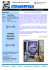More information

### BOCHK AM off to a flying startMore information

### BIRDVILLE INDEPENDENT SCHOOL DISTRICT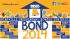More information

### - Dhaka TribuneMore information

### the horizon foundation investing in business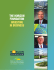More information

### Board presentation on bond - Star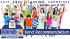More information

### Buy Bond Cars - Free Car MagMore information

### RP2013-3 - Department of Economics and Business EconomicsMore information

### Trustees Call for November 7 Bond Election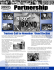More information

### chem 304 inorganic chemistry laboratory manual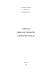More information

### the issue and chart (p15) here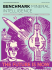More information

### Semi-empirical and ab initio study of the ideal strengths and elastic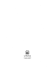More information

### Bank Size, Market Concentration, and Bank Earnings Volatility in theMore information

### Getting Started with DIBELS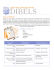More information

### Test Report August 2005 - Principled TechnologiesMore information

### 0 - SMU Digital Collections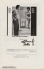More information

### Summer / Fall 2012 - ChristianAudio.com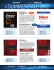More information

### 149.HHS March Newsletter E.inddMore information

### ACQUES TEWART - CommanderBond.net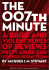More information

### Local Currency Bonds and Infrastructure Finance in ASEAN+3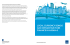More information

### Table of Contents - Options University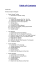More information

### NetworksMore information